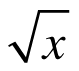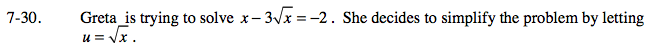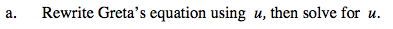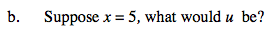### Home > CALC > Chapter 7 > Lesson 7.1.3 > Problem7-30

7-30.
1. Greta is trying to solve x − 3= −2. She decides to simplify the problem by letting u =. Homework Help ✎

1. Rewrite Greta's equation using u, then solve for u.

2. Suppose x = 5, what would u be?$\text{If }u=\sqrt{x},\text{ then }u^{2}=x .$

u2 − 3u = −2
Solve for u.

If u = 2 and u = 1, what is x?Refer to the given information.